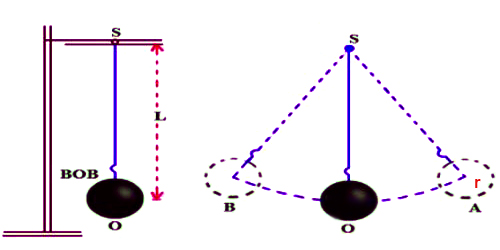Physics

# Determination of the Value of Gravity by the help of Simple PendulumDetermination of the value of Gravity by the help of Simple Pendulum

Theory: The acceleration due to gravity, g at a particular place can be determined by a simple pendulum. For this, the following equation is used.

T = 2π √(L/g)

Here, T = time period, L = effective length and g = acceleration due to gravity.

Now, squaring both sides of the above equation we get,

T2 = 4π2 (L/g)

or, g = 4π2 (L/T2) … …. …. (1)

π is a constant and g is constant at a particular place. So, a particular value of L/T2 will be found at that place and by putting the average value of L/T2 in equation (1), we can determine the value of g.Experiment: At first by a metre scale let the length of the thread of the simple pendulum, l is determined. Then by a slide callipers the diameter of the bob is measured from which the radius ‘r’ of the bob is calculated. The effective length, L = l + r is determined. Now, in the experimental site, the pendulum is allowed to oscillate so that the angular amplitude does not exceed 40. Time t for 20 complete oscillations is taken by a stop-watch. The time period, T = t/20; is found out and square of it is calculated. Now, by changing the length of the pendulum time periods are found out for different effective lengths. T2 is found out in each came.